Data Structures and Algorithms with Object-Oriented Design Patterns in C## Exercises

1.   For each of the following implementations derive an expression for the total memory space required to represent a set which contains of n elements drawn from the universe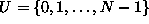.
1. SetAsArray (Program),
2. SetAsBitVector (Program),
3. MultisetAsArray (Program), and
4. MultisetAsLinkedList (Program).
2.   In addition to = and, a complete repertoire of set operators includes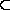,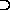,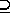and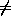. For each of the set implementations listed in Exerciseshow how to implement the remaining operators.
3. The symmetric difference   of two sets S and T, written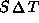is given by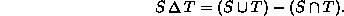For each of the set implementations listed in Exercisedevise an algorithm to compute symmetric difference. What is the running time of your algorithm?

4. The complement  of a set S over universe U, written S' is given by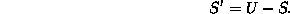Devise an algorithm to compute the complement of a set represented as a bit vector. What is the running time of your algorithm?

5. Devise an algorithm to sort a list of integers using a multiset. What is the running time of your algorithm? Hint: See Section.
6.   Consider a multiset implemented using linked lists. When the multiset contains duplicate items, each of those items occupies a separate list element. An alternative is to use a linked list of ordered pairs of the form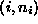where i an the element of the universal set U and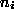is a non-negative integer that counts the number of instances of the element i in the multiset.

Derive an expression for the total memory space required to represent a multiset which contains of n instances of m distinct element drawn from the universe.

7.   Consider a multiset implemented as described in Exercise. Devise algorithms for set union, intersection, and difference. What are the running times of your algorithms?
8.   Consider the initial partition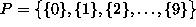. For each of the methods of computing the union listed below show the result of the following sequence join operations:,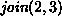,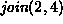,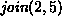,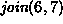,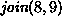,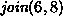,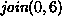,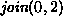.
1. simple union,
2. union by size,
3. union by height, and
4. union by rank.
9. For each final partition obtained in Exercise, show the result of performing a collapsing find operation for item 9.
10. Consider the initial partition P of the universecomprised of N sets.
1. Show that N-1 join operations can be performed before the number of elements in the partition is reduced to one.
2. Show that if n join operations are done (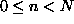), the size of the largest element of the partition is at most n+1.
3. A singleton  is an element of a partition that contains only one element of the universal set. Show that when n join operations are done (), at least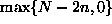singletons are left.
4. Show that if less that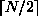join operations are done, at least one singleton is left.Copyright © 2001 by Bruno R. Preiss, P.Eng. All rights reserved.